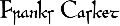All Inscriptions according their Runic Numbers and Values

 a () [ 25 ] * 20 = 500 A (æ) (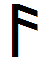) [ 26 ] * 16 = 416 f 1 (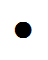) [ 1] * 2 = 2 b (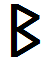) [ 18 ] * 5 = 90 u 2 () [ 2] * 1 = 2 c (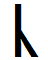) [ 6 ] * 5 = 30 þ 3 (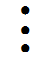) [ 3] * 1 = 3 d (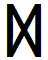) [ 23 ] * 10 = 230 o 4 () [ 4] * 0 = 0 e () [ 19 ] * 19 = 361 E (œ) (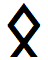) [ 24 ] * 2 = 48 r 5 () [ 5] * 0 = 0 f () [ 1 ] * 12 = 12 g () [ 7 ] * 20 = 140 G () [ 33 ] * 0 = 0 dots * number = value The dots have already been added to the runic values. Sum of all values then 3574 h () [ 9 ] * 9 = 81 i (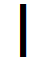) [ 11 ] * 29 = 319 I (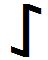) [ 13 ] * 0 = 0 j (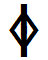) [ 12 ] * 0 = 0 J (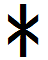) [ 29 ] * 0 = 0 k (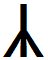) [ 31 ] * 0 = 0 K (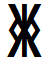) [ 31 ] * 0 = 0 l (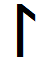) [ 21 ] * 8 = 168 m (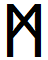) [ 20 ] * 7 = 140 n (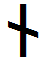) [ 10 ] * 16 = 160 N (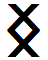) [ 22 ] * 0 = 0 o (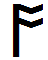) [ 4 ] * 17 = 68 p (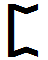) [ 14 ] * 0 = 0 q (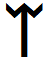) [ 28 ] * 0 = 0 r (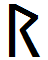) [ 5 ] * 30 = 150 s ([ 16 ] * 20 = 320 S (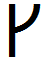) [ 16 ] * 0 = 0 t (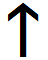) [ 17 ] * 12 = 204 T (þ) (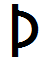) [ 3 ] * 9 = 27 u (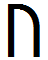) [ 2 ] * 12 = 24 v () [ 2 ] * 0 = 0 w (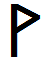) [ 8 ] * 8 = 64 x (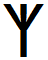) [ 15 ] * 0 = 0 y () [ 27 ] * 1 = 27 z () [ 15 ] * 0 = 0 Z (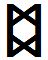) [ 32 ] * 0 = 0

Sum of all Runic Values: 3567 0r 3572 depending on interpretation

 Frequency Rune Value = Sum Dots etc. 1 y27 27 3 x 9 2 E (œ)24 48 2 x 24; 4 x 12 5 b c18, 6 120 (90; 30) 5 x 24; 10 x 12 7 m20 140 7 x 20 8 l w21, 8 232 (168; 64) 29 x 8 9 h þ9, 3 108 (81; 27) 9 x 12 10 d23 230 23 x 10 12 f t u1, 17, 2 240 (12; 204; 24) 10 x 24; 20 x 12 15 æ26 390 26 x 3 x 5 16 n10 160 10 x 16; 20 x 8 17 o4 68 4 x 17 20 a g s e25, 7, 16, 19 1340 (500; 140; 320; 380) 67 x 20 30 i (29?) r11, 5 480 (330; 150) 20 x 24; 40 x 12 288 22 aus 33 runes 302 3567 (or 3572)

As all runes and numbers were supposed to bear magic quality it is difficult to prove magic intent. But numerous patterns are so regularly designed that we can take that as proof.

• First there was the correlation of the symbolic names of the alliterating runes F-, R-, T- und H-Panels) (always at the same spot - upper left corner)with the themes of the pictures.

• Then we counted regular runic numbers with the inscriptions (48 , 72 bzw.74+22). basing on 24. Altogether we counted 288 runes (= 12 x 24). That is 12 times the runic number of the fuÞarks.

• Finally the runic values of the individual panel produced sums divisible by the values of the thematic runes (f, g - r - t - h, s).

• For the entire casket we assume a total value of 3600, that is 12 x 300, which is 12 times the value of the 24 runes in the older fuÞark.

• Actually, the rune master uses the 28 types of the Anglo-Frisian row (value 406), but he makes use of only 22 different runes the value of which is 302. This is close to the value of the old fuÞark, which is 300.

• The list above shows the frequency of appearance of the individual runes on the casket.It may not be surprising that we have 12 times the same number of runes, but rather their values, 4 of which can be divided by 24. Each total seems to be intended. As for an example, we find the 5 always in context with war.

• The symbolic runes of the helpers at war have the value 5 (r) or 15 (x). If theappears 30 times (value 150), then it seems to cite the valkyrian helpers (), 10 times.

• Thus it is just consequent that the æ-rune,, which goes with that archerÆgil, comes up 15times (3 x 5) in the entire text.

• The holy grove (herh) is the place of the ride-and war-helping creatures, as on the panels R and H. If this is meant to be the traditional oak wood then it is hardly accidental that the a-rune, ac (oak) appears 20 times producing the value 500 produziert.One hundred little helpers at war!

• 20 times we find the runes for g (gift), e (horse) and s (sun). For all these runes we have proves symbolic function. On the H-Panel we have a und e in alliteration, and the picture shows, what the runes mean, oak, andhorse. And now we find that they appear in the same number, just like the three times alliterating s in the subsequent line!

• The t-rune (thematic rune) stands for the god Tiw and has the value 17; the o-rune stands for one or more of the Aesir, os, and appears 17 times.

• Other sums base on 3 or 9 (ideally 3 x 3; 3 x 9; 9 x 9). And so we find the fatal h-rune (thematic rune) with its value 9 represented times, value 81; likewise Þ for Þunor with its value 3, which amounts to the effective 3 x 9 = 27.

These first observations bear a certain amount of speculation. Necessarily so. May be the one or other assumption needs correction, but in their total they show that the inscriptions base on complex calculation. And there is more to detect!

 Source: https://www.franks-casket.de Page Top© 2021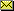Imprint Privacy Policy